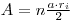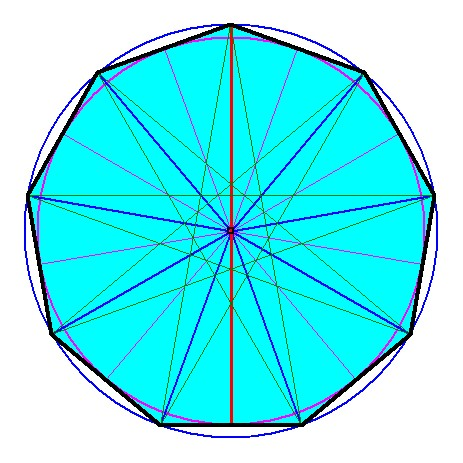Regular Polygon

The angles have the same angle from the center
 Number of angles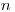Side length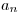Number of anglesSide lengthInterior angle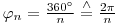Base angle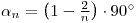Sum of angles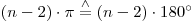Radius of inner circle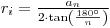Radius of outer circle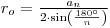Area